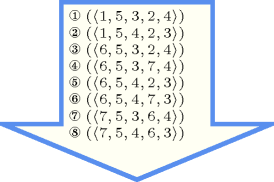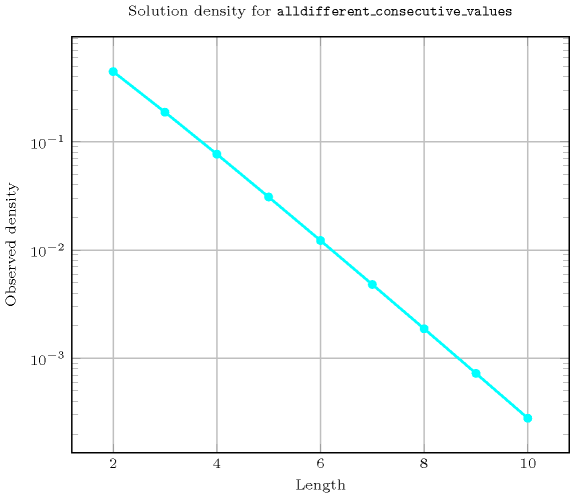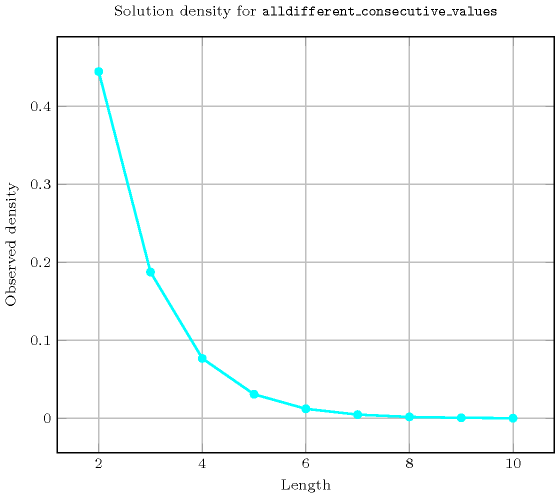## 5.14. alldifferent_consecutive_values

Origin
Constraint

$\mathrm{𝚊𝚕𝚕𝚍𝚒𝚏𝚏𝚎𝚛𝚎𝚗𝚝}_\mathrm{𝚌𝚘𝚗𝚜𝚎𝚌𝚞𝚝𝚒𝚟𝚎}_\mathrm{𝚟𝚊𝚕𝚞𝚎𝚜}\left(\mathrm{𝚅𝙰𝚁𝙸𝙰𝙱𝙻𝙴𝚂}\right)$

Argument
 $\mathrm{𝚅𝙰𝚁𝙸𝙰𝙱𝙻𝙴𝚂}$ $\mathrm{𝚌𝚘𝚕𝚕𝚎𝚌𝚝𝚒𝚘𝚗}\left(\mathrm{𝚟𝚊𝚛}-\mathrm{𝚍𝚟𝚊𝚛}\right)$
Restrictions
 $\mathrm{𝚛𝚎𝚚𝚞𝚒𝚛𝚎𝚍}$$\left(\mathrm{𝚅𝙰𝚁𝙸𝙰𝙱𝙻𝙴𝚂},\mathrm{𝚟𝚊𝚛}\right)$ $\mathrm{𝚊𝚕𝚕𝚍𝚒𝚏𝚏𝚎𝚛𝚎𝚗𝚝}$$\left(\mathrm{𝚅𝙰𝚁𝙸𝙰𝙱𝙻𝙴𝚂}\right)$
Purpose

Enforce (1) all variables of the collection $\mathrm{𝚅𝙰𝚁𝙸𝙰𝙱𝙻𝙴𝚂}$ to take distinct values and (2) constraint the difference between the largest and the smallest values of the $\mathrm{𝚅𝙰𝚁𝙸𝙰𝙱𝙻𝙴𝚂}$ collection to be equal to the number of variables minus one (i.e., there is no holes at all within the used values).

Example
$\left(〈5,4,3,6〉\right)$

The $\mathrm{𝚊𝚕𝚕𝚍𝚒𝚏𝚏𝚎𝚛𝚎𝚗𝚝}_\mathrm{𝚌𝚘𝚗𝚜𝚎𝚌𝚞𝚝𝚒𝚟𝚎}_\mathrm{𝚟𝚊𝚕𝚞𝚎𝚜}$ constraint holds since (1) all the values 5, 4, 3 and 6 are distinct and since (2) all values between value 3 and value 6 are actually used.

All solutions

Figure 5.14.1 gives all solutions to the following non ground instance of the $\mathrm{𝚊𝚕𝚕𝚍𝚒𝚏𝚏𝚎𝚛𝚎𝚗𝚝}_\mathrm{𝚌𝚘𝚗𝚜𝚎𝚌𝚞𝚝𝚒𝚟𝚎}_\mathrm{𝚟𝚊𝚕𝚞𝚎𝚜}$ constraint: ${V}_{1}\in \left\{0,1,3,4,5,6,7,8\right\}$, ${V}_{2}\in \left[4,5\right]$, ${V}_{3}\in \left[3,4\right]$, ${V}_{4}\in \left[0,7\right]$, ${V}_{5}\in \left[3,4\right]$, $\mathrm{𝚊𝚕𝚕𝚍𝚒𝚏𝚏𝚎𝚛𝚎𝚗𝚝}_\mathrm{𝚌𝚘𝚗𝚜𝚎𝚌𝚞𝚝𝚒𝚟𝚎}_\mathrm{𝚟𝚊𝚕𝚞𝚎𝚜}$$\left(〈{V}_{1},{V}_{2},{V}_{3},{V}_{4},{V}_{5}〉\right)$.

##### Figure 5.14.1. All solutions corresponding to the non ground example of the $\mathrm{𝚊𝚕𝚕𝚍𝚒𝚏𝚏𝚎𝚛𝚎𝚗𝚝}_\mathrm{𝚌𝚘𝚗𝚜𝚎𝚌𝚞𝚝𝚒𝚟𝚎}_\mathrm{𝚟𝚊𝚕𝚞𝚎𝚜}$ constraint of the All solutions slotTypical
$|\mathrm{𝚅𝙰𝚁𝙸𝙰𝙱𝙻𝙴𝚂}|>2$
Symmetries
• Items of $\mathrm{𝚅𝙰𝚁𝙸𝙰𝙱𝙻𝙴𝚂}$ are permutable.

• Two distinct values of $\mathrm{𝚅𝙰𝚁𝙸𝙰𝙱𝙻𝙴𝚂}.\mathrm{𝚟𝚊𝚛}$ can be swapped.

• One and the same constant can be added to the $\mathrm{𝚟𝚊𝚛}$ attribute of all items of $\mathrm{𝚅𝙰𝚁𝙸𝙰𝙱𝙻𝙴𝚂}$.

Counting
 Length ($n$) 2 3 4 5 6 7 8 9 10 Solutions 4 12 48 240 1440 10080 80640 725760 7257600

Number of solutions for $\mathrm{𝚊𝚕𝚕𝚍𝚒𝚏𝚏𝚎𝚛𝚎𝚗𝚝}_\mathrm{𝚌𝚘𝚗𝚜𝚎𝚌𝚞𝚝𝚒𝚟𝚎}_\mathrm{𝚟𝚊𝚕𝚞𝚎𝚜}$: domains $0..n$Keywords
Cond. implications

$•$ $\mathrm{𝚊𝚕𝚕𝚍𝚒𝚏𝚏𝚎𝚛𝚎𝚗𝚝}_\mathrm{𝚌𝚘𝚗𝚜𝚎𝚌𝚞𝚝𝚒𝚟𝚎}_\mathrm{𝚟𝚊𝚕𝚞𝚎𝚜}\left(\mathrm{𝚅𝙰𝚁𝙸𝙰𝙱𝙻𝙴𝚂}\right)$

with  $\mathrm{𝚖𝚒𝚗𝚟𝚊𝚕}$$\left(\mathrm{𝚅𝙰𝚁𝙸𝙰𝙱𝙻𝙴𝚂}.\mathrm{𝚟𝚊𝚛}\right)\le 0$

and   $\mathrm{𝚖𝚊𝚡𝚟𝚊𝚕}$$\left(\mathrm{𝚅𝙰𝚁𝙸𝙰𝙱𝙻𝙴𝚂}.\mathrm{𝚟𝚊𝚛}\right)\ge 0$

implies $\mathrm{𝚊𝚖𝚘𝚗𝚐}_\mathrm{𝚍𝚒𝚏𝚏}_\mathtt{0}$$\left(\mathrm{𝙽𝚅𝙰𝚁},\mathrm{𝚅𝙰𝚁𝙸𝙰𝙱𝙻𝙴𝚂}\right)$

when  $\mathrm{𝙽𝚅𝙰𝚁}=|\mathrm{𝚅𝙰𝚁𝙸𝙰𝙱𝙻𝙴𝚂}|-1$.

$•$ $\mathrm{𝚊𝚕𝚕𝚍𝚒𝚏𝚏𝚎𝚛𝚎𝚗𝚝}_\mathrm{𝚌𝚘𝚗𝚜𝚎𝚌𝚞𝚝𝚒𝚟𝚎}_\mathrm{𝚟𝚊𝚕𝚞𝚎𝚜}\left(\mathrm{𝚅𝙰𝚁𝙸𝙰𝙱𝙻𝙴𝚂}\right)$

with  $\mathrm{𝚖𝚒𝚗𝚟𝚊𝚕}$$\left(\mathrm{𝚅𝙰𝚁𝙸𝙰𝙱𝙻𝙴𝚂}.\mathrm{𝚟𝚊𝚛}\right)>0$

implies $\mathrm{𝚊𝚖𝚘𝚗𝚐}_\mathrm{𝚍𝚒𝚏𝚏}_\mathtt{0}$$\left(\mathrm{𝙽𝚅𝙰𝚁},\mathrm{𝚅𝙰𝚁𝙸𝙰𝙱𝙻𝙴𝚂}\right)$

when  $\mathrm{𝙽𝚅𝙰𝚁}=|\mathrm{𝚅𝙰𝚁𝙸𝙰𝙱𝙻𝙴𝚂}|$.

$•$ $\mathrm{𝚊𝚕𝚕𝚍𝚒𝚏𝚏𝚎𝚛𝚎𝚗𝚝}_\mathrm{𝚌𝚘𝚗𝚜𝚎𝚌𝚞𝚝𝚒𝚟𝚎}_\mathrm{𝚟𝚊𝚕𝚞𝚎𝚜}\left(\mathrm{𝚅𝙰𝚁𝙸𝙰𝙱𝙻𝙴𝚂}\right)$

with  $\mathrm{𝚖𝚊𝚡𝚟𝚊𝚕}$$\left(\mathrm{𝚅𝙰𝚁𝙸𝙰𝙱𝙻𝙴𝚂}.\mathrm{𝚟𝚊𝚛}\right)<0$

implies $\mathrm{𝚊𝚖𝚘𝚗𝚐}_\mathrm{𝚍𝚒𝚏𝚏}_\mathtt{0}$$\left(\mathrm{𝙽𝚅𝙰𝚁},\mathrm{𝚅𝙰𝚁𝙸𝙰𝙱𝙻𝙴𝚂}\right)$

when  $\mathrm{𝙽𝚅𝙰𝚁}=|\mathrm{𝚅𝙰𝚁𝙸𝙰𝙱𝙻𝙴𝚂}|$.

$•$ $\mathrm{𝚊𝚕𝚕𝚍𝚒𝚏𝚏𝚎𝚛𝚎𝚗𝚝}_\mathrm{𝚌𝚘𝚗𝚜𝚎𝚌𝚞𝚝𝚒𝚟𝚎}_\mathrm{𝚟𝚊𝚕𝚞𝚎𝚜}\left(\mathrm{𝚅𝙰𝚁𝙸𝙰𝙱𝙻𝙴𝚂}\right)$

implies $\mathrm{𝚋𝚊𝚕𝚊𝚗𝚌𝚎}$$\left(\mathrm{𝙱𝙰𝙻𝙰𝙽𝙲𝙴},\mathrm{𝚅𝙰𝚁𝙸𝙰𝙱𝙻𝙴𝚂}\right)$

when  $\mathrm{𝙱𝙰𝙻𝙰𝙽𝙲𝙴}=0$.

$•$ $\mathrm{𝚊𝚕𝚕𝚍𝚒𝚏𝚏𝚎𝚛𝚎𝚗𝚝}_\mathrm{𝚌𝚘𝚗𝚜𝚎𝚌𝚞𝚝𝚒𝚟𝚎}_\mathrm{𝚟𝚊𝚕𝚞𝚎𝚜}\left(\mathrm{𝚅𝙰𝚁𝙸𝙰𝙱𝙻𝙴𝚂}\right)$

with  $|\mathrm{𝚅𝙰𝚁𝙸𝙰𝙱𝙻𝙴𝚂}|>0$

implies $\mathrm{𝚕𝚎𝚗𝚐𝚝𝚑}_\mathrm{𝚏𝚒𝚛𝚜𝚝}_\mathrm{𝚜𝚎𝚚𝚞𝚎𝚗𝚌𝚎}$$\left(\mathrm{𝙻𝙴𝙽},\mathrm{𝚅𝙰𝚁𝙸𝙰𝙱𝙻𝙴𝚂}\right)$

when  $\mathrm{𝙻𝙴𝙽}=1$.

$•$ $\mathrm{𝚊𝚕𝚕𝚍𝚒𝚏𝚏𝚎𝚛𝚎𝚗𝚝}_\mathrm{𝚌𝚘𝚗𝚜𝚎𝚌𝚞𝚝𝚒𝚟𝚎}_\mathrm{𝚟𝚊𝚕𝚞𝚎𝚜}\left(\mathrm{𝚅𝙰𝚁𝙸𝙰𝙱𝙻𝙴𝚂}\right)$

with  $|\mathrm{𝚅𝙰𝚁𝙸𝙰𝙱𝙻𝙴𝚂}|>0$

implies $\mathrm{𝚕𝚎𝚗𝚐𝚝𝚑}_\mathrm{𝚕𝚊𝚜𝚝}_\mathrm{𝚜𝚎𝚚𝚞𝚎𝚗𝚌𝚎}$$\left(\mathrm{𝙻𝙴𝙽},\mathrm{𝚅𝙰𝚁𝙸𝙰𝙱𝙻𝙴𝚂}\right)$

when  $\mathrm{𝙻𝙴𝙽}=1$.

$•$ $\mathrm{𝚊𝚕𝚕𝚍𝚒𝚏𝚏𝚎𝚛𝚎𝚗𝚝}_\mathrm{𝚌𝚘𝚗𝚜𝚎𝚌𝚞𝚝𝚒𝚟𝚎}_\mathrm{𝚟𝚊𝚕𝚞𝚎𝚜}\left(\mathrm{𝚅𝙰𝚁𝙸𝙰𝙱𝙻𝙴𝚂}\right)$

implies $\mathrm{𝚖𝚊𝚡}_𝚗$$\left(\mathrm{𝙼𝙰𝚇},\mathrm{𝚁𝙰𝙽𝙺},\mathrm{𝚅𝙰𝚁𝙸𝙰𝙱𝙻𝙴𝚂}\right)$

when  $\mathrm{𝙼𝙰𝚇}=$$\mathrm{𝚖𝚊𝚡𝚟𝚊𝚕}$$\left(\mathrm{𝚅𝙰𝚁𝙸𝙰𝙱𝙻𝙴𝚂}.\mathrm{𝚟𝚊𝚛}\right)-\mathrm{𝚁𝙰𝙽𝙺}$.

$•$ $\mathrm{𝚊𝚕𝚕𝚍𝚒𝚏𝚏𝚎𝚛𝚎𝚗𝚝}_\mathrm{𝚌𝚘𝚗𝚜𝚎𝚌𝚞𝚝𝚒𝚟𝚎}_\mathrm{𝚟𝚊𝚕𝚞𝚎𝚜}\left(\mathrm{𝚅𝙰𝚁𝙸𝙰𝙱𝙻𝙴𝚂}\right)$

implies $\mathrm{𝚖𝚒𝚗}_𝚗$$\left(\mathrm{𝙼𝙸𝙽},\mathrm{𝚁𝙰𝙽𝙺},\mathrm{𝚅𝙰𝚁𝙸𝙰𝙱𝙻𝙴𝚂}\right)$

when  $\mathrm{𝙼𝙸𝙽}=$$\mathrm{𝚖𝚒𝚗𝚟𝚊𝚕}$$\left(\mathrm{𝚅𝙰𝚁𝙸𝙰𝙱𝙻𝙴𝚂}.\mathrm{𝚟𝚊𝚛}\right)+\mathrm{𝚁𝙰𝙽𝙺}$.

$•$ $\mathrm{𝚊𝚕𝚕𝚍𝚒𝚏𝚏𝚎𝚛𝚎𝚗𝚝}_\mathrm{𝚌𝚘𝚗𝚜𝚎𝚌𝚞𝚝𝚒𝚟𝚎}_\mathrm{𝚟𝚊𝚕𝚞𝚎𝚜}\left(\mathrm{𝚅𝙰𝚁𝙸𝙰𝙱𝙻𝙴𝚂}\right)$

with  $|\mathrm{𝚅𝙰𝚁𝙸𝙰𝙱𝙻𝙴𝚂}|>0$

implies $\mathrm{𝚖𝚒𝚗}_\mathrm{𝚗𝚟𝚊𝚕𝚞𝚎}$$\left(\mathrm{𝙼𝙸𝙽},\mathrm{𝚅𝙰𝚁𝙸𝙰𝙱𝙻𝙴𝚂}\right)$

when  $\mathrm{𝙼𝙸𝙽}=1$.

$•$ $\mathrm{𝚊𝚕𝚕𝚍𝚒𝚏𝚏𝚎𝚛𝚎𝚗𝚝}_\mathrm{𝚌𝚘𝚗𝚜𝚎𝚌𝚞𝚝𝚒𝚟𝚎}_\mathrm{𝚟𝚊𝚕𝚞𝚎𝚜}\left(\mathrm{𝚅𝙰𝚁𝙸𝙰𝙱𝙻𝙴𝚂}\right)$

with  $\mathrm{𝚖𝚒𝚗𝚟𝚊𝚕}$$\left(\mathrm{𝚅𝙰𝚁𝙸𝙰𝙱𝙻𝙴𝚂}.\mathrm{𝚟𝚊𝚛}\right)=0$

implies $\mathrm{𝚗𝚒𝚗𝚝𝚎𝚛𝚟𝚊𝚕}$$\left(\mathrm{𝙽𝚅𝙰𝙻},\mathrm{𝚅𝙰𝚁𝙸𝙰𝙱𝙻𝙴𝚂},\mathrm{𝚂𝙸𝚉𝙴}_\mathrm{𝙸𝙽𝚃𝙴𝚁𝚅𝙰𝙻}\right)$

when  $\mathrm{𝙽𝚅𝙰𝙻}=\left(|\mathrm{𝚅𝙰𝚁𝙸𝙰𝙱𝙻𝙴𝚂}|+\mathrm{𝚂𝙸𝚉𝙴}_\mathrm{𝙸𝙽𝚃𝙴𝚁𝚅𝙰𝙻}-1\right)/\mathrm{𝚂𝙸𝚉𝙴}_\mathrm{𝙸𝙽𝚃𝙴𝚁𝚅𝙰𝙻}$.

$•$ $\mathrm{𝚊𝚕𝚕𝚍𝚒𝚏𝚏𝚎𝚛𝚎𝚗𝚝}_\mathrm{𝚌𝚘𝚗𝚜𝚎𝚌𝚞𝚝𝚒𝚟𝚎}_\mathrm{𝚟𝚊𝚕𝚞𝚎𝚜}\left(\mathrm{𝚅𝙰𝚁𝙸𝙰𝙱𝙻𝙴𝚂}\right)$

implies $\mathrm{𝚛𝚊𝚗𝚐𝚎}_\mathrm{𝚌𝚝𝚛}$$\left(\mathrm{𝚅𝙰𝚁𝙸𝙰𝙱𝙻𝙴𝚂},\mathrm{𝙲𝚃𝚁},\mathrm{𝚅𝙰𝚁𝙸𝙰𝙱𝙻𝙴𝚂}\right)$

when  $\mathrm{𝙲𝚃𝚁}\in \left[\le \right]$

and   $𝚁=|\mathrm{𝚅𝙰𝚁𝙸𝙰𝙱𝙻𝙴𝚂}|$.

$•$ $\mathrm{𝚊𝚕𝚕𝚍𝚒𝚏𝚏𝚎𝚛𝚎𝚗𝚝}_\mathrm{𝚌𝚘𝚗𝚜𝚎𝚌𝚞𝚝𝚒𝚟𝚎}_\mathrm{𝚟𝚊𝚕𝚞𝚎𝚜}\left(\mathrm{𝚅𝙰𝚁𝙸𝙰𝙱𝙻𝙴𝚂}\right)$

implies $\mathrm{𝚜𝚘𝚏𝚝}_\mathrm{𝚊𝚕𝚕𝚍𝚒𝚏𝚏𝚎𝚛𝚎𝚗𝚝}_\mathrm{𝚌𝚝𝚛}$$\left(𝙲,\mathrm{𝚅𝙰𝚁𝙸𝙰𝙱𝙻𝙴𝚂}\right)$.

Arc input(s)

$\mathrm{𝚅𝙰𝚁𝙸𝙰𝙱𝙻𝙴𝚂}$

Arc generator
$\mathrm{𝑆𝐸𝐿𝐹}$$↦\mathrm{𝚌𝚘𝚕𝚕𝚎𝚌𝚝𝚒𝚘𝚗}\left(\mathrm{𝚟𝚊𝚛𝚒𝚊𝚋𝚕𝚎𝚜}\right)$

Arc arity
Arc constraint(s)
$\mathrm{𝚃𝚁𝚄𝙴}$
Graph property(ies)
$\mathrm{𝐑𝐀𝐍𝐆𝐄}$$\left(\mathrm{𝚅𝙰𝚁𝙸𝙰𝙱𝙻𝙴𝚂},\mathrm{𝚟𝚊𝚛}\right)=|\mathrm{𝚅𝙰𝚁𝙸𝙰𝙱𝙻𝙴𝚂}|-1$